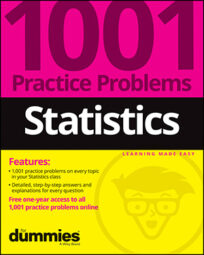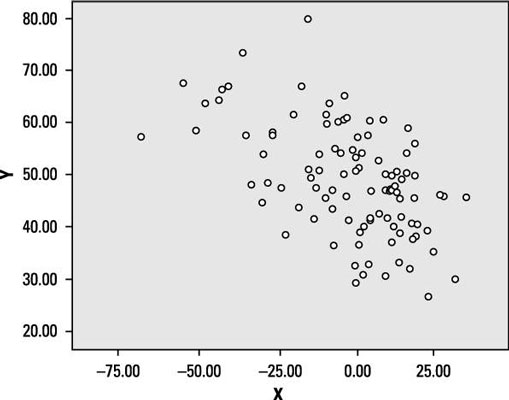##### Statistics: 1001 Practice Problems For Dummies (+ Free Online Practice)Your job here is to find and interpret the results of a regression line and its elements and to carefully check exactly how well your line fits. Note: Regression assumes you've found that a strong relationship exists.

Use the following scatter plot to answer the following problems.Credit: Illustration by Ryan Sneed

## Sample questions

1. In terms of numbers, what is the most plausible value for the correlation between X and Y?

These variables have a moderate negative correlation, as evidenced by their loose clustering around a line running from the upper left to the lower right. In fact, their correlation is –0.54.

2. If the variables X and Y were switched in this scatter plot, how would the correlation be affected?

Correlation measures the strength of the pattern around a line as well as the direction of the line (uphill or downhill). When you switch X and Y, you don't change the strength of their relationship or the direction of the relationship. For example, if the correlation between height and weight is –0.54, the correlation between weight and height is still –0.54.

3. Does the scatter plot suggest that X and Y are good candidates for a linear regression analysis?

Answer: Yes, because they're moderately correlated and the points suggest a linear trend.

The scatter plot indicates a possible linear relationship between the variables, and the correlation coefficient of –0.54 typically has an absolute value high enough to justify beginning a linear regression analysis.

If you need more practice on this and other topics from your statistics course, visit 1,001 Statistics Practice Problems For Dummies to purchase online access to 1,001 statistics practice problems! We can help you track your performance, see where you need to study, and create customized problem sets to master your stats skills.